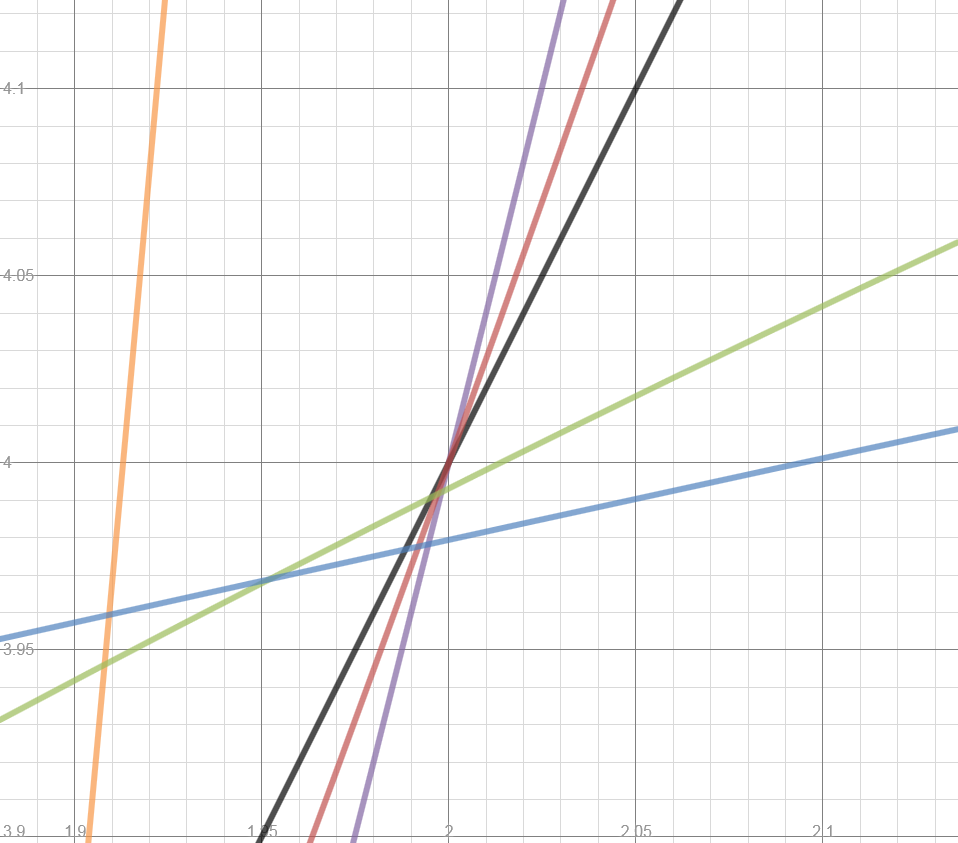Introduction to Derivatives

Posted on 5 commentsOk, let’s do this.  Not saying any of these things are life-changing, but I’m just helping my fellow precal teachers out with some resources. 🙂

Day 1:

Can you guess what type of graphs these are in this desmos file?YOU ARE ALL WRONG.  WRONG WRONG WRONG. BIG FAT DUMMIES.This was the best introduction to tangent lines I’ve done. So much so I’m going to take out the bit about what are and are not tangent lines in the NTM below because they were all “duh, of course those aren’t tangent lines–because it’s not the line the graph would look like if we super-zoomed in!”(they filled in b-g to watch the AROC get closer and closer to 2.)

File here.

Next year, I think I want to make the graph bigger at the bottom, so then we could look at, say, the derivative value when x = 3, then go to desmos and zoom in on the original and see if it looks like a slope of 6.  “Well, wouldn’t that be a handy thing to know just from the derivative graph!”

Day 2:  Modified MathTeacherMambo worksheet.  1) I added a t-chart with specific x-values to use (the first time I did this they couldn’t make smart choices about what points to pick) 2) only did the function to derivative portion and 3) Added this summary at the bottom.

Next year, I’ll do this table instead:I drew this on the board one day a few days into the chapter and BOOM everything made sense.

• The value of f(x) tells us nothing about the derivative value
• We don’t care (yet) about the slope of the derivative graph
• If I know f'(x) is positive, then I know f(x) is increasing
• If I know f(x) is positive, then….uh….I know f(x) is positive.
• WHO CARES WHERE THE DERIVATIVE HAS A MAX OR MIN? Not me.

Day 3: Derivative Match Game

Dude, you have to do this activity, even if you do nothing else in derivative land.  Such a great review of everything and good thinking about derivatives!!  Because I like to sketch on my graph, I made the kiddos a worksheet where they could sketch and record (also helpful if it continues to next day and easier to check).

This is how I like to sketch information about derivatives, as seen in #1 below: Mark your max/mins as boundaries/x-intercepts (blue), determine if graph is increasing/decreasing in each section, determine where derivative should be (yellow), play connect-the-highlighting-and-x-intercepts (red).  Obviously there are some times that you need more thinking than that, but you can get a good starting idea. (This method may be “DUH!” to y’all, but it was a revelation when I walked some kid through it a couple years ago.)File here. (I also changed the cards to be categories instead of D1, etc because “France Pink Otter” is more fun to say)

Day 4: I introduced the derivative function at one point formula which I’m going to skip and let Calculus pick up next year.

Day 5: Let’s derivatize some things!  And see what happens!  And what it could tell us!After they solve #10, have them do the same with ax^2+bx+c and wait for the gasps when you get -b/(2a)!

File here.

I think also next year, I’d like to go back and do the second part of Math Teacher Mambo’s worksheet, except give them f(x) and have them find f'(x) using the formula. Use f'(x) to fill in t-chart from the first worksheet, then use that t-chart to sketch graph of f(x).  Check in calculator.

Day 6:  The derivative workout!   SO MUCH SWEAT!!  (I need to take out question (b)–it just confuses everything, and put question (a) later.)File here.

A day to review and digest everything, then a test that involved using the derivative formula, matching derivative and function graphs, and telling information about f(x) from f'(x). Stay tuned for “Derivatives Part II: You’re Telling Me There was a Shortcut THIS WHOLE TIME?”  (Update: Now posted here!)

Category: Precal

5 comments on “Introduction to Derivatives”

1. […] Part I for Intro to […]

2. Thanks for sharing these! Using several of your worksheets in class this week.

• Hope they were useful! Be sure to check out some more recent stuff on this post: http://www.megcraig.org/?p=1162 🙂

3. Can you please send me the derivative workout file. It looks so creative.

• Sent it to you and also fixed the link in the post. 🙂